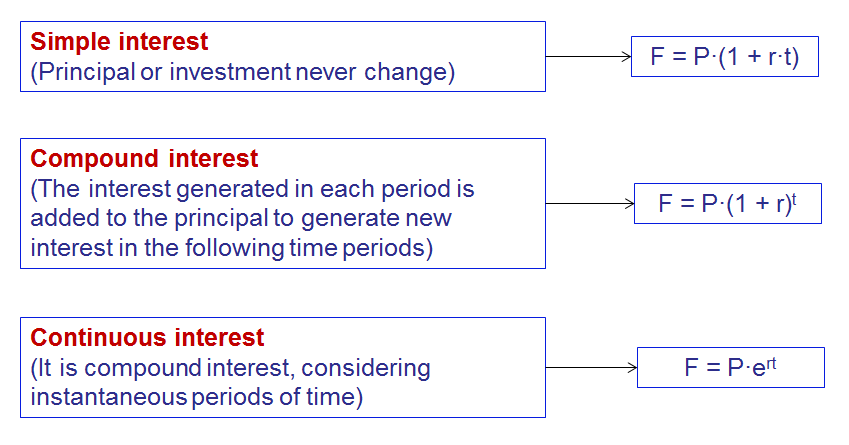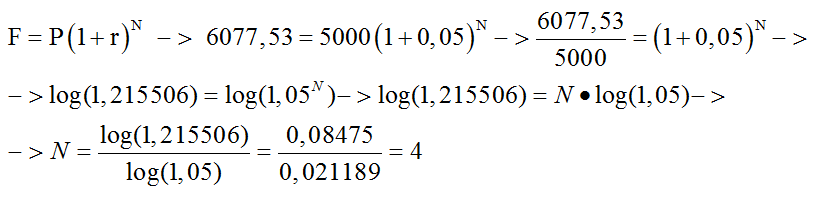# Basic finance concepts

## Simple, compound and continuous interest### Example 1.-

Alex is interested in investing 2000€ during 3 years. Calculate the final amount he will obtain by investing them at an interest rate of 3%, considering simple, compound and continuous interest.

Solution.-

Simple interest:$F = P \cdot (1 + r \cdot t) = 2000(1+0,03 \cdot 3) = 2180,00$

Compound interest:$F = P \cdot (1 + r)^t = 2000 \cdot (1+0,03)^3 = 2185,45$

Continuous interest:$F = P \cdot e^{rt} = 2000 \cdot e^{0,03 \cdot 3} = 2188,35$

### Example 2.-

If you need to have 3000 € at the end of 10 year, how much money do you have to invest today in a product which offers a 12% of simple interest?

Solution.-How much money should you invest if it was compound interest?

Solution.-### Example 3.-

Maria is going to invest today 5000 € in a product which offers a compound interest of 5%. At the end of the period she will received 6077,53€. Calculate the number of periods of this investment?

Solution.-## Periodic rates, nominal rate and Equivalent Annual Rate (EAR)

Interest can be perceived in years, but also in months, quarters or any period of time smaller than the year.

A periodic rate ($i_m$) is a rate for a specified compounding period smaller than the year. m is the number of period of this class that there are in a year.

Examples.-

•$i_{12}$: monthly rate.
•$i_4$: quarterly rate.
•$i_2$:: semiannually rate.

Nominal rate (i)

The nominal rate is an annual rate which could be obtained by multiplying the corresponding periodic rate ($i_m$) by the numbers of compounding periods it has per year (m).

i =$i_m \times m$

Equivalent Annual Rate (EAR) or effective rate

The Equivalent Annual Rate (EAR) or effective rate is an annual rate equivalent to the corresponding periodic rate compounded.

It can be obtained by making equivalent the future amount obtained with both rates, the annual and the periodic:$(1 + r) = (1 + i/m)^m = (1 + i_m )^m \rightarrow r = (1 + i/m)^m -1$

### Example 4.-

Compute the monthly interest rate equivalent to an EAR of 10%:

Solution.-### Example 5.-

Compute the nominal rate and the EAR equivalents to 2% of quarterly interest.

Solution.-

Nominal rate:$i = i_4 \cdot 4 = 0,02 \cdot 4 = 0,08 = 8 \%$

Equivalent Annual Rate:$(1 + r)^1=(1 + i_4)^4 \rightarrow (1 + r) = (1 + 0,02)^4 \rightarrow r =(1+0,02)^4 -1 = 0,0824 = 8,24 \%$